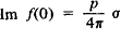# Optical Theorem

Also found in: Wikipedia.
The following article is from The Great Soviet Encyclopedia (1979). It might be outdated or ideologically biased.

## Optical Theorem

a theorem that establishes a connection between the decrease in intensity of a wave propagating through a medium and the total scattering cross section of the wave. The optical theorem was initially formulated in physical optics and expressed the imaginary part of the index of refraction in terms of the total scattering cross section of the light-scattering centers, or oscillators. This imaginary part describes the absorption of light.

In quantum mechanics the optical theorem is the result of the unitary condition, which requires that the total probability of all the possible processes occurring in a system be equal to unity, and relates the imaginary part of the forward elastic scattering amplitude I m f(0) to the total cross section cr of the scattering of the particle by a center of force or by another particle:where ρ is the momentum of the incident particle in the center-of-mass system. The optical theorem is used in establishing a connection between directly measurable scattering characteristics of particles.

V. P. PAVLOV